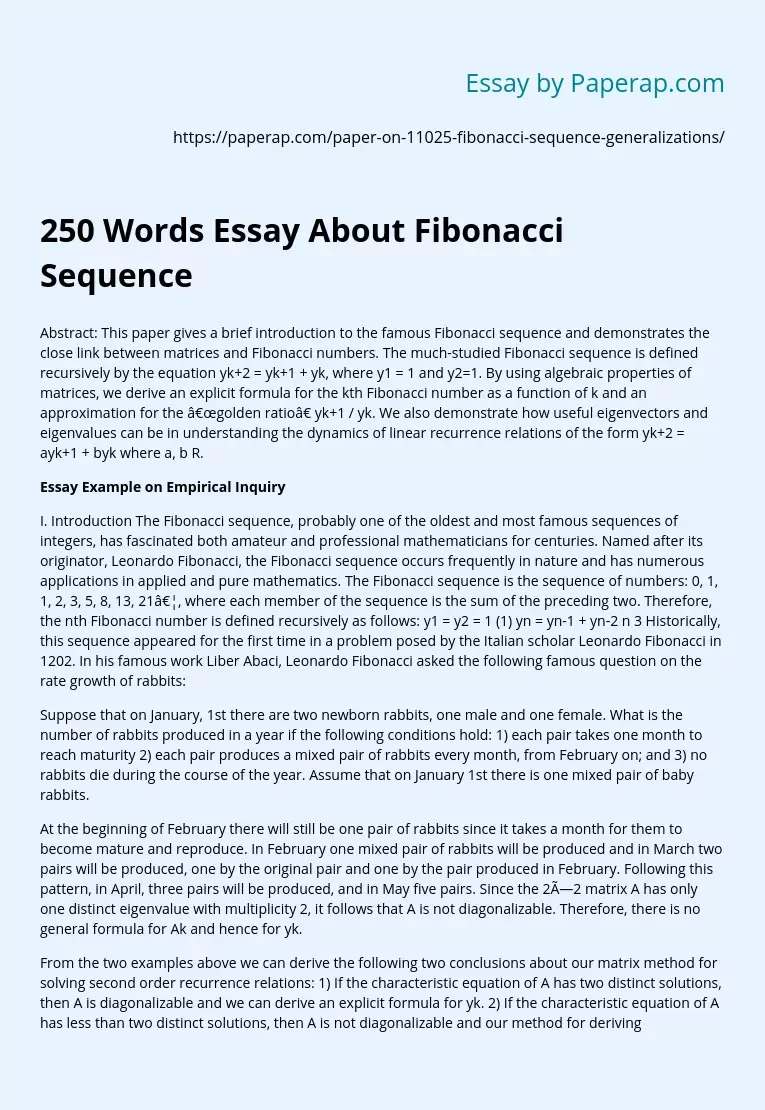# 250 Words Essay About Fibonacci Sequence

Abstract: This paper gives a brief introduction to the famous Fibonacci sequence and demonstrates the close link between matrices and Fibonacci numbers. The much-studied Fibonacci sequence is defined recursively by the equation yk+2 = yk+1 + yk, where y1 = 1 and y2=1. By using algebraic properties of matrices, we derive an explicit formula for the kth Fibonacci number as a function of k and an approximation for the “golden ratio” yk+1 / yk. We also demonstrate how useful eigenvectors and eigenvalues can be in understanding the dynamics of linear recurrence relations of the form yk+2 = ayk+1 + byk where a, b R.

## Essay Example on Empirical Inquiry

I. Introduction The Fibonacci sequence, probably one of the oldest and most famous sequences of integers, has fascinated both amateur and professional mathematicians for centuries. Named after its originator, Leonardo Fibonacci, the Fibonacci sequence occurs frequently in nature and has numerous applications in applied and pure mathematics. The Fibonacci sequence is the sequence of numbers: 0, 1, 1, 2, 3, 5, 8, 13, 21…, where each member of the sequence is the sum of the preceding two.

Therefore, the nth Fibonacci number is defined recursively as follows: y1 = y2 = 1 (1) yn = yn-1 + yn-2 n 3 Historically, this sequence appeared for the first time in a problem posed by the Italian scholar Leonardo Fibonacci in 1202. In his famous work Liber Abaci, Leonardo Fibonacci asked the following famous question on the rate growth of rabbits:

Suppose that on January, 1st there are two newborn rabbits, one male and one female. What is the number of rabbits produced in a year if the following conditions hold: 1) each pair takes one month to reach maturity 2) each pair produces a mixed pair of rabbits every month, from February on; and 3) no rabbits die during the course of the year.

Get quality help nowRhizManVerified

Proficient in: Algebra4.9 (247)

“ Rhizman is absolutely amazing at what he does . I highly recommend him if you need an assignment done ”+84 relevant experts are online

Assume that on January 1st there is one mixed pair of baby rabbits.

At the beginning of February there will still be one pair of rabbits since it takes a month for them to become mature and reproduce. In February one mixed pair of rabbits will be produced and in March two pairs will be produced, one by the original pair and one by the pair produced in February. Following this pattern, in April, three pairs will be produced, and in May five pairs. Since the 2×2 matrix A has only one distinct eigenvalue with multiplicity 2, it follows that A is not diagonalizable. Therefore, there is no general formula for Ak and hence for yk.

From the two examples above we can derive the following two conclusions about our matrix method for solving second order recurrence relations: 1) If the characteristic equation of A has two distinct solutions, then A is diagonalizable and we can derive an explicit formula for yk. 2) If the characteristic equation of A has less than two distinct solutions, then A is not diagonalizable and our method for deriving yk cannot be applied. More formally, our conclusions are consistent with the following more general theorem:

Theorem: Let be the distinct solutions of the equation, where and b = 0. Then every solution of the linear homogenous recurrence relation with constant coefficients, where a0 = C0 and a1 = C1, is of the form for some constants A and B.1 IV. Conclusion This paper discussed how linear algebra can be applied to the analysis of linear recurrence relations, a number of which arise frequently in applied and pure math. In particular, it emphasized the concepts of eigenvalues and eigenvectors and how helpful they are in describing and understanding infinite sequences of integers.

Bibliography:

1 For the proof of this theorem see Koshy, p.143-4